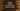# Python program to multiply two float values using a function## Python program to multiply two floating numbers using a function:

In this post, we will learn how to multiply two floating point numbers using a separate function. We will write one separate function that will take the numbers as parameters and return the multiplication value.

If we use a function, we can put all reusable code in that function and call that function from different parts of the program.

A function can take any number of values as parameters and it can return a result to the caller. For this example, the function will take two floating point numbers as its parameters and it will return the product of these two numbers.

### Python program to multiply two floating numbers using a function:

Let’s take a look at the below program:

``````def multiply(first_no, second_no):
return first_no*second_no

print(multiply(10.0, 2.0))
print(multiply(15.23, 12.98))``````

It will print the below output:

``````20.0
197.68540000000002``````

Here,

• multiply is a function that takes two numbers as parameters and returns its multiplication
• The print statements are calling the multiply function with two different floating point numbers for each.

### Python program to multiply two floating numbers using a function and user input numbers:

We can also take the numbers as user inputs. Similar to the above program, we can call the function with the user input values and it will print the result.

``````def multiply(first_no, second_no):
return first_no*second_no

first = float(input('Enter the first number :'))
second = float(input('Enter the second number :'))

print('{}*{} = {}'.format(first, second, multiply(first, second)))``````

It will print output as like below:

``````Enter the first number :12.5
Enter the second number :11.6
12.5*11.6 = 145.0

Enter the first number :12.3
Enter the second number :14.67
12.3*14.67 = 180.441``````

Here,

• we are using input to take the number as input from the user.
• But input reads the value as string. So, we are passing that value to float() to convert it to a floating point value.